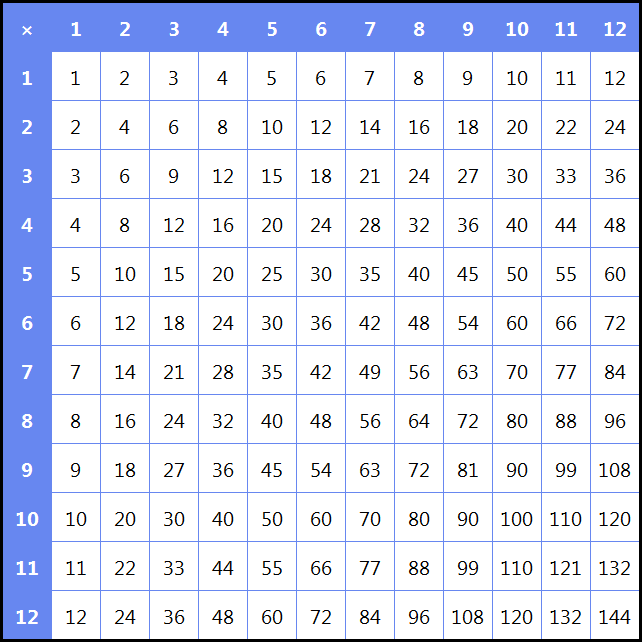﻿ ToTable – Algosim documentation
Algosim documentation: ToTable

# ToTable

Converts an object to a table.

## Syntax

• `ToTable(X)`

• `X` is any object

## Description

If `X` is any object, `ToTable(X)` returns `X` as a table. Specifically:

• If `X` is a two-dimensional container, like a matrix or a pixmap, then `ToTable(X)` is the table with entries being the elements of `X` converted to strings using the `string` function.

• If `X` is a string, then it is split into rows and columns by splitting on CRLF and TAB, respectively.

• If `X` is a two-dimensional nested list, then `ToTable(X)` returns the table with entries being the second-level elements of `X` converted to strings using the `AsSingleLine` function.

• If non of the above applies and `X` is a one-dimensional container (such as a vector or a list), then `ToString(X)` is the column table consisting of the entries obtained from `X` by means of `string`.

• Otherwise, the result is a single-cell (1×1) table with text `string(X)`.

## Examples

```T ≔ compute(a⋅b, a, 1, 12, b, 1, 12);
for(i, 1, 12, VarInsert(T[i], 1, i));
VarInsert(T, 1, SequenceList(0, 12));
T ≔ "×";
display(ToTable(T))
```